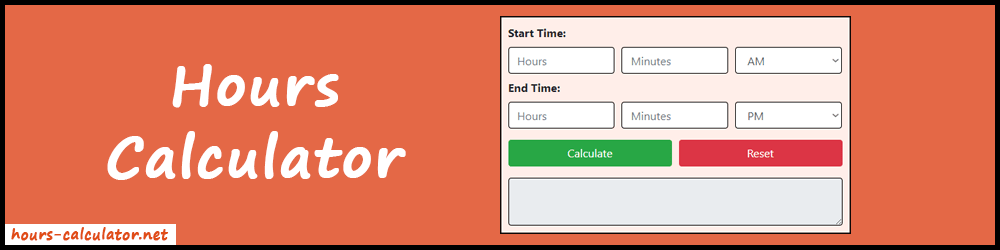# Hours Calculator

Hours calculator helps to find the number of hours and minutes between starting time and ending time. Also, it gives total minutes.

For a full time card, use the Time Card Calculator.

##### More Useful Tools
Birthday Calculator

Hours calculator is a free tool that computes duration between two sets of times in hours and minutes. It's also known as Time Duration Calculator. It's very easy to operate. Just enter the starting and ending time and press the calculate button to get the final result.

In short, it's very useful as well as handy when it comes to calculating hours and minutes between two times. Moreover, you can find the days between dates using the date calculator.This online tool allows you to manually enter the time from where you want to start and end the calculation. Then the algorithm behind the calculator gives accurate duration results in hours and minutes.

Now, forget the old ways of finding the time duration. This tool is the one-stop destination for all your time calculations. Furthermore, the process is very simplified for easy and fast usage.

## Why Use the Hours Calculator?

There are many real-life uses of this calculator. From personal usage to business applications, it can be effectively used anywhere.

• Manage Personal Time Card: Using our tool you can easily manage and update your personal time card. For example, you can calculate the time period elapsed during some activity. Also, you can check how many hours you have worked on any task or project. Lastly, you can use those hours to make the weekly timesheet. But we recommend using the time card calculator for more ease.
• Payroll Management: Any business can use this tool to manage their payroll effectively. Also, it will save your time and effort.
• Countdown: If you are planning something and want to know the time left, you can use it to know how much the time left.

There are many more purposes for that you can use this calculator to make your work effortless.

### How to Calculate Hours Between Two Times?

A simple arithmetic operation is involved to calculate the hours between two times. Let's understand it with an example.

#### Example:

Find out the hours and minutes between following times:

Start time = 10:05 AM
End time = 12:30 PM

#### Solution:

Firstly, subtract the starting time hours from the ending time hours.
12 - 10 = 2

Similarly, subtract the starting time minutes from the ending time minutes.
30 - 5 = 25

Now, you have the hours and minutes result. So, combine them to get the final result.

Duration = 2 Hours 25 Minutes

1 Hour = 60 Minutes

So, Total Minutes = 60 + 60 + 25 = 145

The same calculation process is running behind the hours calculator. But the complete process takes less than a second. So, use our tool rather than doing a manual calculation.

### How to Use the Hours Calculator?

To use the calculator, follow the steps below.

1. Firstly, set the start and end time by filling the respective input boxes. Either you can manually type the hours and minutes or use the up-down buttons to input the values. Also, don't forget to specifies whether it is "AM" or "PM".
2. Now press the Calculate button to display the final results in hours and minutes. Moreover, it will show you the total minutes too.
3. Lastly, use the Reset option for the new calculation or vacant the input fields.

### FAQs

There is no usage limit. You can use it unlimited times.

Our tool supports only a 12-hour time format. So, make sure the time you have entered is in the proper 12-hours format. Otherwise, it will give you an error message.

Enter the current time in the start time and set midnight time in the end time. As a result, it will show you the time remains on your birthday.

Yes, of course, you can use it on your mobile or iPad. It's mobile and iPad responsive. Also, it works fine on any type of devices.